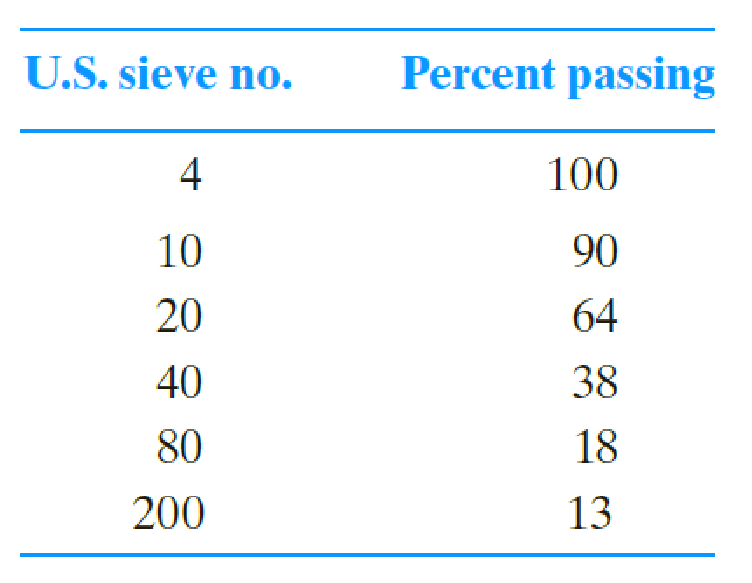Chapter 5, Problem 5.6PPrinciples of Geotechnical Enginee...

9th Edition
Braja M. Das + 1 other
ISBN: 9781305970939

Solutions

Chapter
SectionPrinciples of Geotechnical Enginee...

9th Edition
Braja M. Das + 1 other
ISBN: 9781305970939
Textbook Problem

For an inorganic soil, the following grain-size analysis is given.For this soil, LL = 23 and PL = 19. Classify the soil by using a. AASHTO soil classification system. Give the group index. b. Unified soil classification system. Give group symbol and group name.

a)

To determine

Find the classification of the given soil using AASTHO soil classification.

Explanation

Calculation:

Refer the given data.

The percent of soil passing through U.S.sieve no. 200 is 13 %.

The percent of soil passing through U.S.sieve no. 40 is 38 %.

The percent of soil passing through U.S.sieve no. 10 is 90 %.

Determine the plasticity index (PI) using the formula.

PI=LLPL

Substitute 23 for LL and 19 for PL.

PI=2319=4

Determine the group index of soil A using the formula.

GI=(F20035)[0.2+0.005(LL40)]+0.01(F20015)(PI10)

Here, PL is the plastic limit and LL is the liquid limit.

Substitute 13 for F200, 23 for LL, and 4 for PI.

GI=(1335)[0.2+0.005(2340)]+0

b)

To determine

Find the classification of the given soil using unified soil classification system.

Still sussing out bartleby?

Check out a sample textbook solution.

See a sample solution

The Solution to Your Study Problems

Bartleby provides explanations to thousands of textbook problems written by our experts, many with advanced degrees!

Get Started

Find more solutions based on key concepts